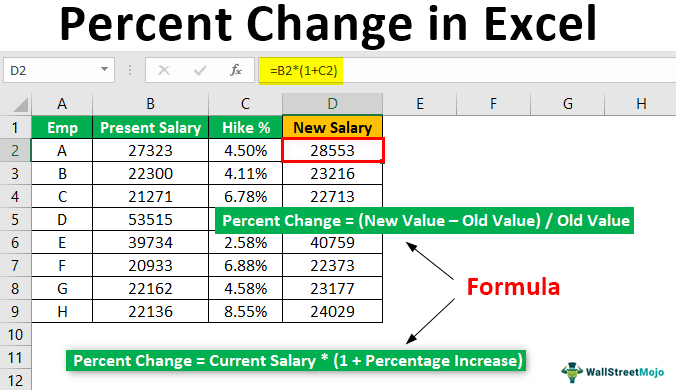Blog

# How to Find Percent Change in Excel?

Are you looking to make quick and accurate calculations of percent change in Excel? Percent change is a useful metric for tracking the difference between two figures over time. It is often used in business to measure the success of a new product, the efficiency of a process, or the effectiveness of a marketing campaign. With this guide, you’ll learn how to find percent change in Excel and get the most out of your data.## How to Calculate Percent Change in Excel?

Excel is a powerful tool for performing calculations. It can be used to quickly and accurately calculate percent change in a variety of ways. Percent change is a measure of how much a value has changed over a period of time. Knowing how to calculate percent change in Excel can help you make better decisions and understand trends in your data.

Calculating percent change in Excel is relatively straightforward. All you need to do is enter the data you want to calculate the change for into a worksheet, then use a formula to calculate the percentage change. Excel provides several formulas that can be used to calculate percentage change. Once you understand the formulas and the data you need to use them, calculating percent change in Excel is a quick and easy task.

### Understanding Percent Change

Before diving into the formulas used to calculate percent change in Excel, it’s important to understand exactly what percent change is. In its simplest form, percent change is the difference between two values divided by the original value. This difference is then expressed as a percentage. For example, if the original value is 5 and the new value is 10, the percent change would be 100%.

It’s important to note that percent change is not the same as a percentage increase or decrease. A percentage increase or decrease is the amount by which a value has increased or decreased relative to the original value, while percent change is the difference between two values divided by the original value. When calculating percent change in Excel, you will need to use the correct formula for the specific calculation you need to make.

### Using the Percent Change Formula

The most common formula used to calculate percent change in Excel is the Percent Change formula. This formula is used to calculate the percentage change between two values. To use the Percent Change formula, you will need to enter the original value in one cell and the new value in another cell. Then, select the cell where you want to display the percent change and enter the following formula:

=ABS(-)/

This formula will calculate the absolute value of the difference between the two values, then divide it by the original value to get the percentage change.

### Calculating Percent Change Over Time

It’s also possible to calculate percent change over a period of time in Excel. To do this, you will need to enter the original value in one cell and the new value in another cell. Then, select the cell where you want to display the percent change and enter the following formula:

=ABS(-)/*100

This formula will calculate the absolute value of the difference between the two values, then divide it by the original value and multiply it by 100 to get the percentage change over a period of time.

### Using Conditional Formatting for Percent Change

You can also use Excel’s conditional formatting feature to quickly identify changes in data. To use this feature, select the data you want to format and click the Conditional Formatting button on the Home tab. Then, select the Percent Change option from the list of formatting rules and select the cells you want to format. Excel will automatically format the cells based on the percentage change you entered.

### Using Graphs to Visualize Percent Change

Finally, you can use Excel’s graphing features to visualize and analyze percent changes over time. To do this, simply enter your data into a worksheet and select the cells you want to graph. Then, click the Insert tab and select the graph type you want to use. Excel will automatically create a graph that displays the percent changes in your data.

By understanding how to calculate percent change in Excel and using the available formulas and features, you can quickly and accurately analyze and visualize your data.